Home > CCA2 > Chapter 9 > Lesson 9.2.1 > Problem9-57

9-57.
1. If a = 2 + 3i and b = 1 − i, compute each of the following operations: Homework Help ✎

1. a + b

2. ab

3. ab

4.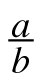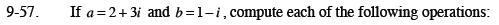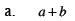(2 + 3i) + (1 − i)
Combine like terms.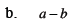1 + 4i
Did you remember to distribute the negative?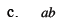A generic rectangle can be used to multiply.
Remeber that i2 = −1.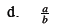Multiply both the numerator and denominator by the complex conjugate of the denominator.

$-\frac{1}{2}+\frac{5}{2}i$